# How much data to you need?

The use of pre-trained language models is beginning to revolutionise applied NLP. Here I present some simple tests on language model and classification accuracy with and without pre-trained language models.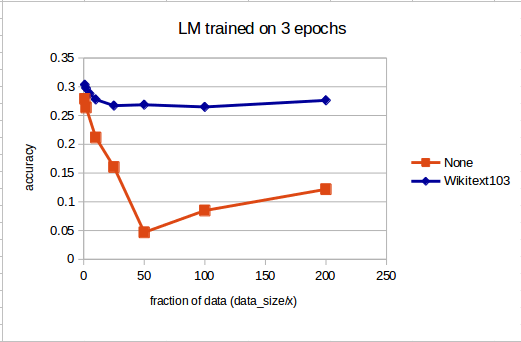Fig. 1. Comparison of accuracy of language model on pre-trained vs non pre-trained model.

In Figure 1 we can see the resulting accuracy of a LSTM RNN Encoder/Decoder language model for the imdb dataset with 100,000 texts in total. The blue line shows accuracy for a model pre-trained on the Wikitext 103 dataset and the orange curve shows results with pretraining. The x axis shows the decimation fraction of the data used for training. Eg for x=10, one tenth of the data ie 10,000 text units was used. Of the total number of text units used for each test, 90% were used for training and 10% used for testing.

The base code for the language model can be found here.

We can see that when the full dataset is used the wikitext pre-trained model performs slightly better than the non pre-trained model. However as the amount of data used for training decreases, the non pre-trained model decreases in accuracy rapidly, while the pre-trained model hits a plateau exhibiting a baseline language model performance essentially without any additional training data.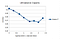Fig.2. Accuracy vs dataset decimation for pre-trained Wikitext 103 language model

In Figure 3 we see a zoom in of Figure 1 for the performance of the pre-trained Wikitext 103 language model on the imdb dataset. At c. 1/25th of the data (total of 4000 texts, of which 90% are used for training) we see a plateau in accuracy reached, below which a baseline accuracy of .26 to .27 is achieved on predicting the next word in a sentence.

For multi-class classification tasks I used the same architecture as per the imdb fastai code.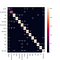Fig. 3. Multi-class classification confusion matrix for pre-trained wikitext-103 language model on the dbpedia dataset — the baseline.

A baseline classification was run using a wikitext 103 pre trained model then trained on 90% of the dbpedia dataset (567,000 samples) to generate the confusion matrix show in Figure 4.

Then a series of tests were made using a pre-trained wiki 103 language model on fractions of the dbpedia dataset used for training. The average F1 score of resulting classifications is shown against the number of training samples in Figure 4 below.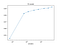Fig. 4. Average F1 score for all classes vs number of samples for classification results for the dbpedia dataset

Where:

`F1 Score = 2*((Precision * Recall) / (Precision + Recall))Recall = True Positives / (True Positives + False Negatives)Precision = True Positives / (True Positives + False Positives)`

We can see that even with a very low number of training samples (630) we are still acheiving a respectable average F1 score (average of f1 score for each class) of ~0.94.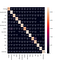Fig 5. Multi-class classification confusion matrix for pre-trained wikitext-103 language model on the dbpedia dataset trained on 1/1000th of the dataset.

The confusion matrix for classification results trained on just 1/1000th of the dataset is shown above.

The tests were repeated for the ag_news dataset (4 classes, 127600 total samples).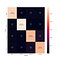Fig. 6. Multi-class classification confusion matrix for pre-trained wikitext-103 language model on the ag_news dataset — the baseline.

Again the average F1 score with different training dataset sizes is shown, here for the ag_news dataset.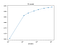Fig. 7. Average F1 score for all classes vs number of samples for classification results for the ag_news dataset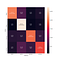Fig 8. Multi-class classification confusion matrix for pre-trained wikitext-103 language model on the ag_news dataset trained on 1/1000th of the dataset.

The main take away from this work is that even with small datasets in the hundreds to few thousands of sample size range, one can get good results by using a pre-trained language model and subsequent fine tuning on a domain specific dataset.

Geophysicist and Deep Learning Practitioner